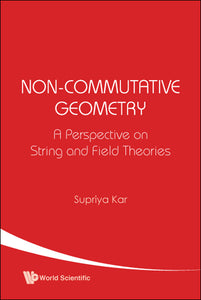# Non-commutative Geometry: A Perspective On String And Field Theories

Supriya K Kar

Format: Print Book

ISBN: 9789812380524

• SGD 66.34
Unit price per
Tax included.

This book provides a systematic, comprehensive and up-to-date account of the recent developments in non-commutative geometry, at a pedagogical level. It does not go into the details of rigorous (advanced level) mathematical formulation of non-commutative geometry; rather, it restricts itself to the domain of strings and quantum fields.Since non-commutative geometry has recently aroused renewed interest in open string theory, the author motivates the text from the viewpoint of a string theory. He begins with an introduction to the subject, explaining what one means by non-commutative geometry and why it is relevant to study such geometry, and discussing its possible origin in a string theory.The book comprises nine chapters. Chapter 1 gives a sound mathematical ntroduction to non-commutative spacetime coordinates in classical and quantum physics. In Chapter 2, non-commutativity in a string theory is discussed at a pedagogic level. Chapter 3 deals with an aribitrary D-brane dynamics and Chapter 4 describes the non-commutative gauge theories on a D-brane. In Chapters 5-9, non-commutative quantum field theory (NCQFT) is addressed. In particular, Chapter 5 deals with the real scalar NCQFT, Chapter 6 with that of complex scalar field, Chapter 7 describes spontaneous symmetry breaking in scalar NCQFT, Chapter 8 deals with the U(1) Gauge theory and Chapter 9 with SU(n) Gauge theories.Students will find this book useful as a bridge between string and field theories. In addition, it will prove invaluable for interdisciplinary areas of study.

Format: Hardcover
No of Pages: 250
Imprint: World Scientific
Publication date: 20210930